#### How to Build a Retirement Calculator in Excel

Thanks to Excel, you can easily calculate things. Here you have a free retirement calculator to find out how much you will get as a senior.

## Preparation of data for calculating the pension

1. Open Excel and make four rows which are periodic deposit, times per year, current age, retirement age, interest rate, number of years to retirement, and total number of deposits per year.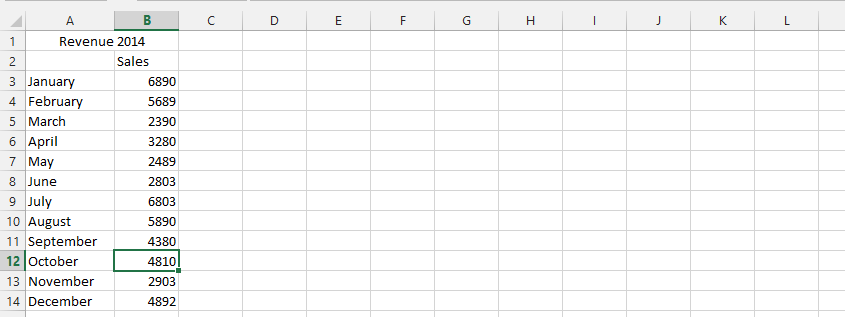2. Enter your data in the cells corresponding to the headings: periodic deposit, times per year, current age, retirement age, and interest rate.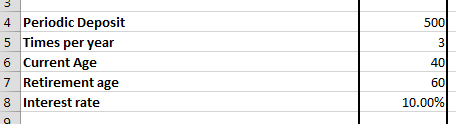## Calculating the pension

3. Calculate the number of years to retirement and the total number of deposits per year. The number of years to retirement is calculated by deducting the current age from the retirement age.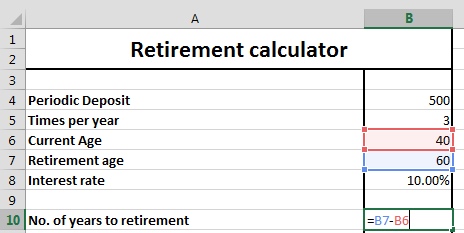4. To calculate the total amount of deposit, simply multiply the number of times per year by the number of years.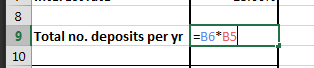5. Now calculate estimated savings using the FV function in Excel in the cell corresponding to estimated savings. The formula goes as follows:

=PV(interest rate/times per year, total number of deposits per year, periodic deposits)*-1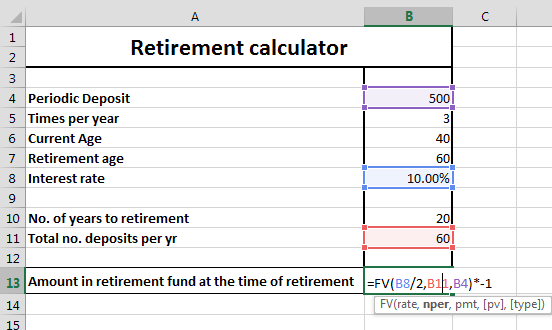The full formula of the retirement calculator is:

=FV(B8/2,B11,B4)*-1